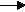gcsescience.com                                       22                                       gcsescience.com

Moles

Volumes of Gases.

Hydrogen is produced in the laboratory
by adding zinc to dilute sulfuric acid.

Example 3.

What volume of hydrogen is produced
by reacting 6·5 g  of zinc with dilute sulfuric acid?

Method.
1) Find how many moles of zinc are present in 6·5 g  of zinc.
RAM of  Zn = 65.

moles = mass ÷ RAM

moles = 6·5 ÷ 65

= 0·1 moles of zinc.

2) Write the equation for the reaction.

zinc    +   sulfuric acidzinc sulfate   +   hydrogen.
Zn(s) +    H2SO4(aq)ZnSO4(aq)      +        H2(g)

Use the big numbers to find the proportion of reactant to product.

1Zn makes 1H2, the proportion is 1 to 1,
so 0·1 moles of zinc will make 0·1 moles of hydrogen.

3) Convert moles into volume.

volume moles x 24,000 cm3

volume 0·1 x 24,000 cm3
= 2,400 cm3 of hydrogen.

So, reacting 6·5 g  of zinc with dilute sulfuric acid
will produce 2,400 cm3 of hydrogen.

gcsescience.com       The Periodic Table       Index       Moles Quiz       gcsescience.com